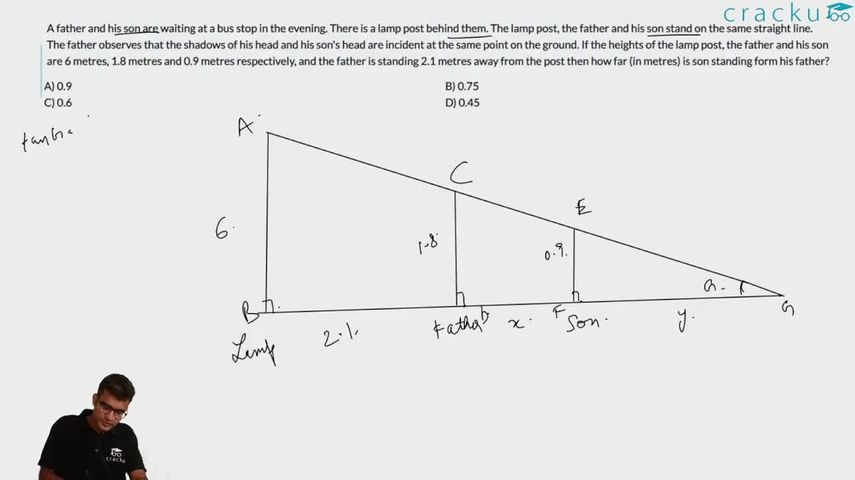Question 18

# A father and his son are waiting at a bus stop in the evening. There is a lamp post behind them. The lamp post, the father and his son stand on the same straight line. The father observes that the shadows of his head and his son's head are incident at the same point on the ground. If the heights of the lamp post, the father and his son are 6 metres, 1.8 metres and 0.9 metres respectively, and the father is standing 2.1 metres away from the post then how far (in metres) is son standing form his father?

Solution

The three triangles are similar.

Let the distance of the tip of the shadow from the child be y. Let the child be standing at distance x from the father.

So, 6/(2.1+x+y) = 1.8/(x+y) = 0.9/y

=> 2y = x+y => x = y

=> 6/(2.1 + 2x) = 0.9/x

=> 6x = 0.9*(2.1+2x)

=> 6x = 1.89 + 1.8x

=> 4.2x = 1.89

=> x = 1.89/4.2 = 0.45### View Video Solution